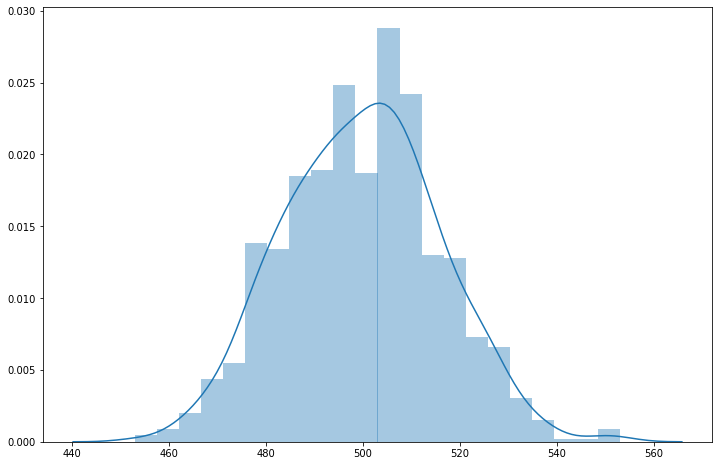# Coin flipping¶

The ideas behind flipping a coin are important in computing.

Computers rely on bits - a bit is a variable that can take on one of two values, one or zero.

Flipping a coin results in one of two outcomes, heads or tails.

In :
# We'll use numpy and scipy.stats to analyse flipping a coin.
import numpy as np
import scipy.stats as ss

# We'll use this for visualisation.
import matplotlib.pyplot as plt
import seaborn as sns

In :
# This just sets the default plot size to be bigger.
plt.rcParams['figure.figsize'] = (12, 8)


A fair coin will give a head fifty percent of the time and a tail fifty percent of the time.

We say the probability of a head is 0.5 and the probability of a tail is 0.5.

We can use the following function to simulate this - giving a 1 for a head and 0 for a tail.

In :
# (Number of times to flip a coin, probability of a head, number of times to do this)
np.random.binomial(1, 0.5, 1) # returns the head(1) or tail(0) value

Out:
array()
In :
# Flip a fair coin 1000 times - how many heads?
np.random.binomial(1000, 0.5, 1) # returns the number of heads

Out:
array()

How likely are we to see a certain number of heads when flipping a coin however many times?

In :
# (No. of heads, no. of flips, probability of a head)
ss.binom.pmf(500, 1000, 0.5) # ss - scipy stats -

Out:
0.025225018178380496
In :
sns.distplot(np.random.binomial(1000, 0.5, 1000))

Out:
<matplotlib.axes._subplots.AxesSubplot at 0x22dfd7d52e8>In :
# Flip an unfair coin 10 times - how many heads?
np.random.binomial(10, 0.2, 1)

Out:
array()

Suppose we flip an unfair coin ($p = 0.3$) ten times, what is the probability that the flips are as follows?

$$HHTTHHHTTT$$
In :
(0.3)*(0.3)*(1.0-0.3)*(1.0-0.3)*(0.3)*(0.3)*(0.3)*(1.0-0.3)*(1.0-0.3)*(1.0-0.3)

Out:
0.0004084101
In :
0.3*0.3*0.7*0.7*.3*.3*.3*.7*.7*.7

Out:
0.0004084101

The probability of $r$ heads when flipping an unfair coin $n$ times is

$$P(r \mid n , p) = {n \choose r} p^r (1-p)^{(n-r)}$$
$${n \choose r} = \frac{n!}{r!(n-r)!}$$$${10 \choose 3} = \frac{10!}{3!(7)!}$$$$= 120$$$$\therefore P = {120} (0.3^3)(0.7^7)$$$$= 0.266$$
In :
(0.3)**5*(0.7)**5

Out:
0.00040841009999999976
In :
noflips = 10

p = 0.3
d = [ss.binom.pmf(i, noflips, p) for i in range(noflips+1)]
d

Out:
[0.028247524900000005,
0.12106082100000018,
0.2334744405,
0.26682793200000016,
0.20012094900000013,
0.10291934520000007,
0.03675690899999999,
0.009001692000000002,
0.0014467004999999982,
0.00013778100000000015,
5.904899999999995e-06]

${n \choose r}$ is the number of ways to select $r$ items from $n$, ignoring the order you select them in.

In :
import math

n = 10
r = 3

choose = lambda x, y: math.factorial(x) / (math.factorial(y) * math.factorial(x-y))

choose(n, r)

Out:
120.0

Note the following for ${n \choose 0}$ and ${n \choose n}$.

In :
choose(10, 0)

Out:
1.0
In :
choose(n, n)

Out:
1.0
In :
choose(10,3)

Out:
120.0
In :
choose(8,4)

Out:
70.0

Even though the chances are, with $p = 0.3$ and $10$ flips, that there are three heads, the most probable outcome is all tails.

Clarification: The one instance of tails beats a single instance of three heads, not all 120 possible options of three heads.

In :
(1-0.3)**10

Out:
0.02824752489999998
In :
0.7**10

Out:
0.02824752489999998
In :
0.3**10

Out:
5.9048999999999975e-06
In :
0.7**9*0.3**1

Out:
0.012106082099999993

What has all of this got to do with computers and bits?

Would you consider the following a data set?

In :
import itertools
#["".join(seq) for seq in itertools.product("01", repeat=10)]